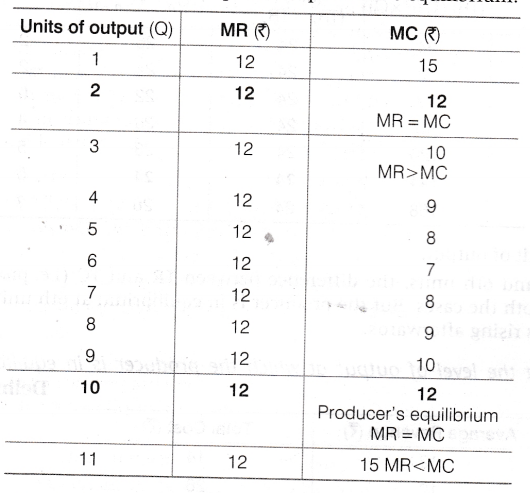# Explain the conditions of producer's equilibrium with the help of a numerical example

Producer’s equilibrium refers to a situation, where a producer is producing that level of output, at which its profits are maximum. In other words, it is a situation of profit maximisation or cost minimisation (under MR and MC approach).
Following schedule explain the producer’s equilibrium:Conditions of producer’s equilibrium
Following are the two conditions of producer’s equilibrium:
(i) MR=MC (Marginal Revenue = Marginal Cost)
(ii) MC must be rising at the point of equilibrium or MC curve must cut MR curve from below.
In the given schedule MR = MC, both at 2 units and 10 units of output, but the second condition of rising MC is fulfilled only at 10th unit of output. So, the producer is in equilibrium when he is producing 10 units.